# Application of Derivatives NCERT Solutions for Class 12 Maths Chapter 6

In this page we have Application of Derivatives NCERT Solutions for Class 12 Maths Chapter 6 for EXERCISE 6.2 . Hope you like them and do not forget to like , social share and comment at the end of the page.

Question 1
Show that the function given by f(x) = 3x + 17 is strictly increasing on R.
Solution
f(x) = 3x + 17
Differentiating w.r.t x
f'(x) = 3 > 0, in every interval of R.
Thus, the function is strictly increasing on R.

Question 2
Show that the function given by f(x) = e2x  is strictly increasing on R.
Solution
f(x) = e2x
Differentiating w.r.t x
f'(x) =2e2x > 0, in every interval of R.
Hence, f is strictly increasing on R.

Question 3
Show that the function given by f(x) = sin x is
(a) strictly increasing in ( 0 ,π/2)
(b) strictly decreasing in ( π/2, π)
(c) neither increasing nor decreasing in (0, π)
Solution
The given function is f(x) = sin x.
Differentiating w.r.t x
f'(x) = cosx
(a) Since for each ( 0  ,π/2) we have  cos x > 0
Hence, f is strictly increasing in ( 0 ,π/2)
(b) Since for each ( π/2, π)  , we have  cos x < 0
Hence, f is strictly decreasing in ( π/2, π)
(c) From the results obtained in (a) and (b), it is clear that f is neither increasing nor
decreasing in (0, π).

Question 4
Find the intervals in which the function f given by f(x) = 2x2− 3x is
(a) strictly increasing (b) strictly decreasing
Solution
f(x) = 2x2− 3x
Differentiating w.r.t x
f'(x) =4x-3
Equating f'(x) =0, we get
4x-3 =0
x= ¾
The point x = 3/4 divides the real line into two disjoint intervals, namely, (–∞ , 3/4), (3/4, ∞)
In interval (– ∞, 3/4), f'(x) < 0
Hence, the given function (f) is strictly decreasing in interval (– ∞, 3/4)
In interval (3/4, ∞) , f'(x) > 0
Hence, the given function (f) is strictly increasing in interval (3/4, ∞)

Question 5
Find the intervals in which the function f given by f(x) = 2x3− 3x2 − 36x + 7 is
(a) strictly increasing (b) strictly decreasing
Solution
f(x) = 2x3− 3x2 − 36x + 7
Differentiating w.r.t x
f'(x) =6x2-6x-36
Equating f'(x) =0, we get
6x2-6x-36=0
Or  x = − 2, 3
The points x = −2 and x = 3 divide the real line into three disjoint intervals i.e., (– ∞, -2), (-2, 3) and ( 3, ∞)
In intervals is positive (– ∞, -2), (3, ∞), f'(x) >  0
while in interval (−2, 3), is negative, f'(x) < 0
Hence, the given function (f) is strictly increasing in intervals (– ∞, -2), (3, ∞)
, while function (f) is strictly decreasing in interval (−2, 3),

Question 6
Find the intervals in which the following functions are strictly increasing or decreasing:
(a) x2+ 2x − 5
(b) 10 − 6x − 2x2
(c) −2x3− 9x2− 12x + 1
(d) 6 − 9x – x2
(e) (x + 1)3(x − 3)3
Solution
(a) We have,
F(x) = x2+ 2x – 5
Differentiating w.r.t x
f'(x) =2x+2
Equating f'(x) =0, we get
2x+2=0
x = −1
Point x = −1 divides the real line into two disjoint intervals i.e., (– ∞, -1) and (-1, ∞)
In interval (– ∞, -1), f'(x) < 0
f is strictly decreasing in interval (– ∞, -1)
In interval (-1,∞ ), f'(x) > 0
f is strictly increasing in interval (-1,∞ )
(b) We have,
f(x) = 10 − 6x − 2x2
Differentiating w.r.t x
f'(x) =-6-4x
Equating f'(x) =0, we get
-6-4x=0
x=-3/2
The point divides the real line into two disjoint intervals i.e., (– ∞, -3/2) and (-3/2, ∞)
In interval (– ∞, -3/2), f'(x) > 0
f is strictly increasing for (– ∞, -3/2)
In interval (-3/2,∞), f'(x) < 0
f is strictly decreasing for (-3/2,∞)
(c) We have,
f(x) = −2x3− 9x2− 12x + 1
Differentiating w.r.t x
f'(x) =-6x2-18x-12 =-6(x+1) (x+2)
Equating f'(x) =0, we get
-6x2-18x-12=0
x2 +3x +2 =0
(x+1)(x+2) =0
Or x=-1, -2
Points x = −1 and x = −2 divide the real line into three disjoint intervals i.e., (– ∞, -2), (-2, -1) and ( -1, ∞)
In intervals, i.e., when x < −2 and x > −1, f'(x) < 0
f is strictly decreasing for x < −2 and x > −1.
Now, in interval (−2, −1) i.e., when −2 < x < −1, f'(x) > 0
f is strictly increasing for −2 < x < −1
(d) We have,
F(x) =6 − 9x – x2
Differentiating w.r.t x
f'(x) =-9-2x
Equating f'(x) =0, we get
x= -9/2
The point divides the real line into two disjoint intervals i.e., (– ∞, -9/2) and (-9/2, ∞)
In interval (– ∞, -9/2), f'(x) > 0
f is strictly increasing for (– ∞, -9/2)
In interval (-9/2, ∞) , f'(x) < 0
f is strictly decreasing for (-9/2, ∞)
(e) We have,
f(x) = (x + 1)3(x − 3)3
Differentiating w.r.t x
f'(x)  =3(x + 1)2(x − 3)3 +3(x + 1)3(x − 3)2
=3(x+1)2( x-3)2 [ x-3 + x+1]
=6(x+1)2( x-3)2 (x-1)
Equating f'(x) =0, we get
x = -1 or 3 or 1
The points x = −1, x = 1, and x = 3 divide the real line into four disjoint intervals
i.e. (– ∞, -1), (−1, 1), (1, 3), and (3, ∞)
In intervals and (−1, 1), (– ∞, -1), f'(x) < 0
f is strictly decreasing in intervals and (−1, 1).
In intervals (1, 3) and (3, ∞), f'(x) > 0
f is strictly increasing in intervals (1, 3) and (3, ∞)

Question 7
Show that y = log(1+x) -2x/(2+x) x > 1 is an increasing function of x throughout its
domain.
Solution
We have,
y = log(1+x) -2x/(2+x)
Differentiating w.r.t x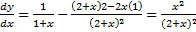Equating dy/dx =0, we get
x2 /(2+x)2 =0
or x =0 as x >-1
Since x > −1, point x = 0 divides the domain (−1, ∞) in two disjoint intervals i.e., −1 <
x < 0 and x > 0.
When −1 < x < 0, we have dy/dx > 0
Also, when x > 0, we have dy/dx > 0
Hence, function f is increasing throughout this domain.

Question 8
Find the values of x for which y = [x (x – 2)]2   is an increasing function.
Solution
We have,
y = [x (x – 2)]2
y= (x2 -2x)2 = x4 +4x2 -4x3
Differentiating w.r.t x
dy/dx = 4x3 +8x- 12x2
Equating dy/dx =0, we get
4x (x2 +2-3x) =0
4x(x-1) (x-2) =0
The points x = 0, x = 1, and x = 2 divide the real line into four disjoint intervals i.e.,
( -∞,0),(0,1),( 1,2) (2,∞)
In intervals ( -∞,0),(1,2)  , dy/dx < 0
y is strictly decreasing in intervals, dy/dx
However, in intervals (0, 1) and (2, ∞), dy/dx > 0
y is strictly increasing in intervals (0, 1) and (2, ∞).

Question 9
Prove that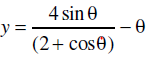is an increasing function of θ in [0, π/2]
Solution
We have,Differentiating w.r.t q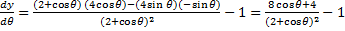Equating dy/d θ =0, we get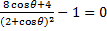8 cos q + 4 = (2+ cos q)2
8 cos q + 4 =4+ 4cos q + cos 2 q
Cos2 q -4cos q=0
Cos q( cos q -4)
Since cos θ ≠ 4, cos θ = 0.
Or q= π/2
Now,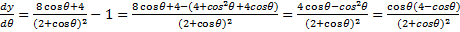In interval (0, π/2) we have cos θ > 0. Also, 4 > cos θ, 4 − cos θ > 0
So dy/dq > 0
Therefore, y is strictly increasing in interval (0, π/2)
Also, the given function is continuous at 0 and π/2
Hence, y is increasing in interval [0, π/2]

Question 10
Prove that the logarithmic function is strictly increasing on (0, ∞).
Solution
F(x) = log x
Differentiating w.r.t x
f'(x) =1/x
It is clear that for x > 0, f'(x) > 0
Hence, f(x) = log x is strictly increasing in interval (0, ∞).

Question 11
Prove that the function f given by f(x) = x2− x + 1 is neither strictly increasing nor strictly decreasing on (−1, 1).
Solution
f(x) = x2− x + 1
Differentiating w.r.t x
f'(x) =2x -1
Equating f'(x) =0, we get
2x-1 = 0
x= 1/2
The point divides the interval (−1, 1) into two disjoint intervals (-1, ½) and (½,1)
Now, in interval (-1, ½), f'(x) =2x -1   < 0
Therefore, f is strictly decreasing in interval (-1, ½)
However, in interval (½,1), f'(x) =2x -1   > 0
Therefore, f is strictly increasing in interval (½,1)
Hence, f is neither strictly increasing nor decreasing in interval (−1, 1).

Question 12
Which of the following functions are strictly decreasing on (0, π/2)?
(A) cos x (B) cos 2x (C) cos 3x (D) tan x
Solution
(A) Let f(x) =cos x
f’(x) = -sin x
In interval (0, π/2), f’(x) < 0
So, f(x) is strictly decreasing in interval (0, π/2)
(B) Let
F(x) = cos 2x
f’(x) = -2 sin 2x
for x in (0, π/2), 2x would in (0, π), So sin2x > 0
and f’(x) = -2 sin 2x < 0
So, cos 2x is strictly decreasing in interval (0, π/2)
(C) Let f(x) = cos 3x
f’(x) = -3 sin 3x
Equating f’(x)=0
Sin 3x =0
Or x = π/3 as x in (0, π/2)
The point divides the interval into two disjoint intervals (0, π/3) and (π/3, π/2)
For interval (0, π/3), f’(x) = -3 sin 3x < 0
So, it is strictly decreasing in interval (0, π/3)
For interval (π/2, π/3), f’(x) = -3 sin 3x > 0
So, it is strictly increasing in interval (π/2, π/3),
Hence, function is neither increasing nor decreasing in interval (0, π/2)
(D) Let
f(x) = tan x
f’(x) = sec2 x
for interval (0, π/2), f’(x) > 0
So, function is strictly increasing in interval (0, π/2)
Therefore, functions cos x and cos 2x are strictly decreasing in (0, π/2)
Hence, the correct answers are A and B.

Question 13
On which of the following intervals is the function f given by f(x) = x100 + sinx -1 strictly
decreasing?
(A) (0,1)
(B) (π/2, π)
(C) (0, π/2)
(D) None of these
Solution
We have,
f(x) = x100 + sinx -1
f’(x) = 100x99 +cos x
In interval (0,1), f’(x) > 0 as cos x > 0 and 100x99 > 0
Thus, function f is strictly increasing in interval (0, 1).
In interval (π/2, π), cos x < 0 and 100x99 > 0 and 100x99 > cos x, So f’(x) > 0
Thus, function f is strictly increasing in interval (π/2, π)
In interval (0, π/2), cos x > 0 and 100x99 > 0, So f’(x) > 0
f is strictly increasing in interval (0, π/2)
Hence, function f is strictly decreasing in none of the intervals.

Question 14
Find the least value of a such that the function f given f(x) = x2 + ax + 1 is strictly increasing on (1, 2).
Solution
We have,
f(x) = x2 + ax + 1
f’(x) =2x+a
Now, function f will be increasing in (1, 2), if in (1, 2), f’(x) > 0
2x + a > 0
2x > −a
x > -a/2
Now x lies in (1,2), So the least value of a such that
-a/2 =1
a=-2
Hence, the required value of a is −2.

Question 15
Let I be any interval disjoint from (−1, 1). Prove that the function f given by
F(x) = x +1/x    is strictly increasing on I.
Solution
f(x) = x +1/x
f’(x) =1 -1/x2
1- 1/x2 =0
x2 =1
or x =+1, -1
The points x = 1 and x = −1 divide the real line in three disjoint intervals i.e., (- ∞, -1) ,( -1, 1) and (1, ∞)
In interval (−1, 1), it is observed that
x2 < 1
1/x2 > 1
Or 0 > 1 -1/x2 (x ≠ 0)
f is strictly decreasing on (−1, 1)
In intervals (- ∞, -1) and (1, ∞), it is observed that:
x2 > 1
1/x2 < 1
1 – 1/x2 > 0
f is strictly increasing on (- ∞, -1) and (1, ∞)
Hence, function f is strictly increasing in interval I disjoint from (−1, 1).
Hence, the given result is proved.

Question 16
Prove that the function f given by f(x) = log sin x is strictly increasing on (0, π/2)
And strictly decreasing on (π/2, π)
Solution
f(x) = log sin x
f’(x)  = (1/sin x) cos x = cot x
In interval (0, π/2), f’(x) =cot x > 0
f is strictly increasing in (0, π/2)
In interval (π/2, π), f’(x) =cot x < 0
f is strictly decreasing in (π/2, π)

Question 17
Prove that the function f given by f(x) = log cos x is strictly decreasing on (0, π/2)
And strictly increasing on (π/2, π)
Solution
f(x) = log cos x
f’(x) = (1/cos x) (-sin x) = -tan x
In interval (0, π/2), f’(x) = -tan x < 0
f is strictly decreasing on (0, π/2)
In interval (π/2, π), f’(x) = -tan x > 0
f is strictly increasing on (π/2, π)

Question 18
Prove that the function given by f(x) = x3 – 3x2 + 3x – 100 is increasing in R.
Solution
f(x) = x3 – 3x2 + 3x – 100
f’(x) = 3x2 – 6x + 3= 3(x-1)2
For any x in R, (x − 1)2 > 0.
Hence, the given function (f) is increasing in R.

Question 19
The interval in which y = x2 e-x   is increasing is
(A) (– ∞, ∞)
(B) (−2, 0)
(C) (2, ∞)
(D) (0, 2)
Solution
y = x2 e-x
dy/dx = 2x e-x – x2 e-x = xe-x( 2-x)
for dy/dx=0
x= 0 or x =2
The points x = 0 and x = 2 divide the real line into three disjoint intervals
i.e., ( -∞,0) , (0 ,2) and ( 2, ∞)
In intervals ( -∞,0) ,( 2, ∞)  ,  dy/dx < 0
f is decreasing on ( -∞,0) ,( 2, ∞) ,
In interval (0, 2), dy/dx > 0
f is strictly increasing on (0, 2).
Hence, f is strictly increasing in interval (0, 2).

## Related Topics

Go back to Class 12 Main Page using below links